# Skip Counting Q2

0%
0Skip Counting - Quiz #2

1 / 10

1.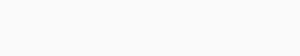Which one is the correct sequence of counting by 9s?

2 / 10

2.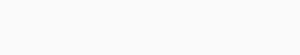Which one is the correct sequence of counting by 15s?

3 / 10

3.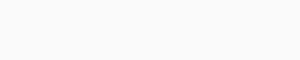Which one is the correct sequence of counting by 8s?

4 / 10

4.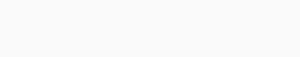Which one is the correct sequence of counting by 11s?

5 / 10

5.Which one is the correct sequence of counting by 7s?

6 / 10

6.Which one is the correct sequence of counting by 9s?

7 / 10

7.Which one is the correct sequence of counting by 8s?

8 / 10

8.Which one is the correct sequence of counting by 10s?

9 / 10

9.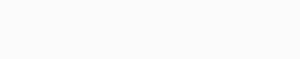Which one is the correct sequence of counting by 8s?

10 / 10

10.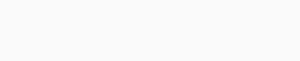Which one is the correct sequence of counting by 9s?